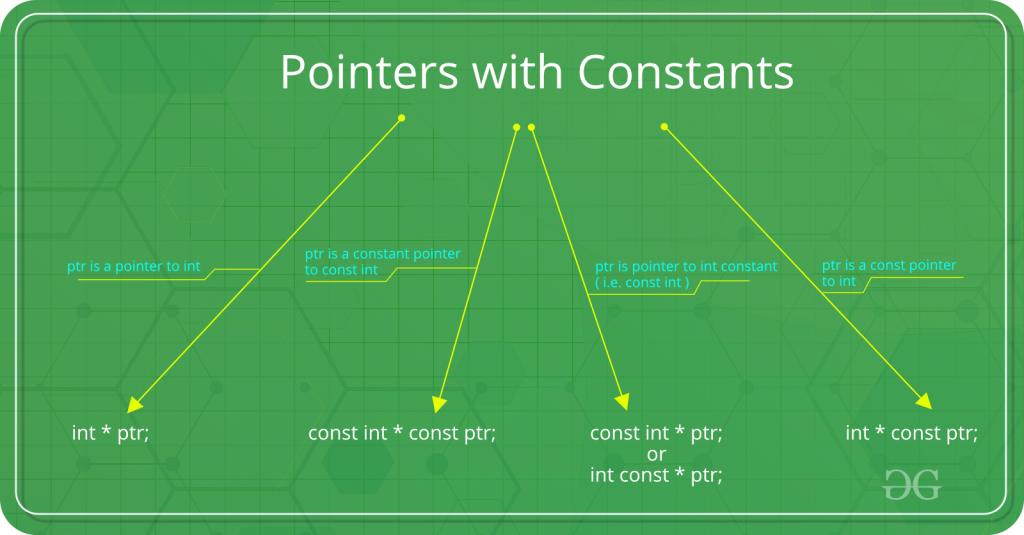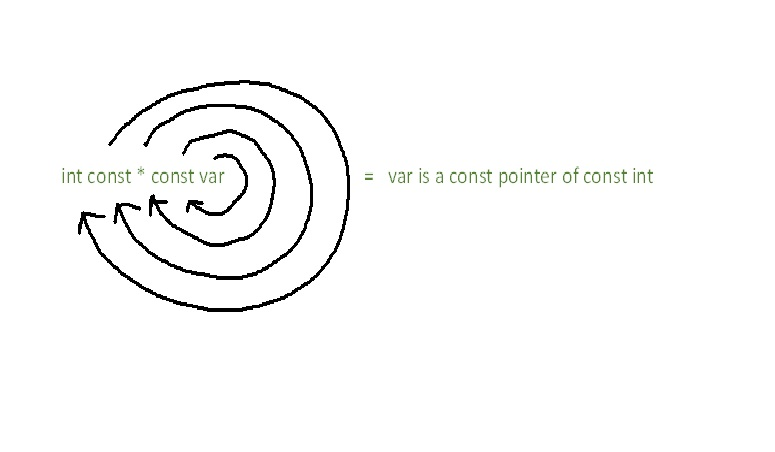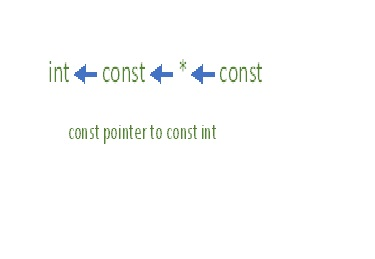# Difference between const int*, const int * const, and int const *## int const*

int const* is pointer to constant integer
This means that the variable being declared is a pointer, pointing to a constant integer. Effectively, this implies that the pointer is pointing to a value that shouldn’t be changed. Const qualifier doesn’t affect the pointer in this scenario so the pointer is allowed to point to some other address.
The first const keyword can go either side of data type, hence int const* is equivalent to const int*.

 `#include ` ` `  `int` `main(){ ` `    ``const` `int` `q = 5; ` `    ``int` `const``* p = &q; ` ` `  `    ``//Compilation error ` `    ``*p = 7; ` ` `  `    ``const` `int` `q2 = 7; ` ` `  `    ``//Valid ` `    ``p = &q2;  ` `     `  `    ``return` `0; ` `} `

## int *const

int *const is a constant pointer to integer

This means that the variable being declared is a constant pointer pointing to an integer. Effectively, this implies that the pointer shouldn’t point to some other address. Const qualifier doesn’t affect the value of integer in this scenario so the value being stored in the address is allowed to change.

 `#include ` ` `  `int` `main(){ ` `    ``const` `int` `q = 5; ` `    ``//Compilation error ` `    ``int` `*``const` `p = &q; ` ` `  `    ``//Valid ` `    ``*p = 7;  ` ` `  `    ``const` `int` `q2 = 7; ` ` `  `    ``//Compilation error ` `    ``p = &q2;  ` ` `  `    ``return` `0; ` `} `

## const int* const

const int* const is a constant pointer to constant integer

This means that the variable being declared is a constant pointer pointing to a constant integer. Effectively, this implies that a constant pointer is pointing to a constant value. Hence, neither the pointer should point to a new address nor the value being pointed to should be changed.
The first const keyword can go either side of data type, hence const int* const is equivalent to int const* const.

 `#include ` ` `  `int` `main(){ ` `    ``const` `int` `q = 5; ` ` `  `    ``//Valid ` `    ``const` `int``* ``const` `p = &q; ` ` `  `    ``//Compilation error ` `    ``*p = 7; ` `     `  `    ``const` `int` `q2 = 7; ` ` `  `    ``//Compilation error ` `    ``p = &q2; ` `     `  `    ``return` `0; ` `} `

## Memory Map

One way to remember the syntax (according to Bjarne Stroustrup) is the spiral rule-
The rule says, start from the name of the variable and move clockwise to the next pointer or type. Repeat until expression ends.The rule can also be seen as decoding the syntax from right to left.Hence,

• int const* is pointer to const int
• int *const is const pointer to int
• int const* const is const pointer to const int

Using this rule, even complex declarations can be decoded like,

• int ** const is a const pointer to pointer to an int.
• int * const * is a pointer to const pointer to an int.
• int const ** is a pointer to a pointer to a const int.
• int * const * const is a const pointer to a const pointer to an int.

Attention reader! Don’t stop learning now. Get hold of all the important DSA concepts with the DSA Self Paced Course at a student-friendly price and become industry ready.

My Personal Notes arrow_drop_upCheck out this Author's contributed articles.

If you like GeeksforGeeks and would like to contribute, you can also write an article using contribute.geeksforgeeks.org or mail your article to contribute@geeksforgeeks.org. See your article appearing on the GeeksforGeeks main page and help other Geeks.

Please Improve this article if you find anything incorrect by clicking on the "Improve Article" button below.